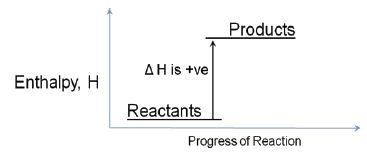# Endothermic Reaction

If the potential energy diagram shows that the energy of the reactants is lower than that of the products, the reaction will take in energy. ΔH is positive. It will be an endothermic reaction. Most chemical reactions involve the exchange of energy between molecules and compounds. The total energy of a chemical reaction is determined from the bonds broken and formed. A tremendous amount of energy is held in the bonds that hold molecules together.Example: One example of an endothermic reaction is: 2NH4 NO3(s) + Ba(OH)2.8H2O(s) + 2NH3 (g) + 10 H2O (I) + Ba(NO3)2 (aq), ΔH = +17.44 kJ mol-1.

Enthalpy and Enthalpy Change: Obviously, these amounts of heat energy released or absorbed must be dependent on the quantity of substances reacting so we must define the enthalpy changes in terms of energy changes (kilo joules) per amount of substance (mole). Therefore, the units of enthalpy and enthalpy change are kJ mol-1.